[中文] Search Internet Search HelloDBA
Oracle Technology Site. email: fuyuncat@gmail.com  MSN: fuyuncat@hotmail.com# Full Table Scan cost formula cracking(3)

[中文]

Author:  fuyuncat

Source:  www.HelloDBA.com

Date:  2009-08-01 15:03:35

Share to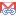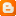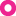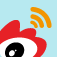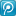### 3.4.4.Filter column position

In fact, this can not be considered separately, because there may be a number of filter columns, each filter column has its own location. We first consider the case of only one filter column.

select /*+full(a)*/a, b, c, d, e from T_PEEKING11 a where col < :v2;

TABROWS: 1000000; TABBLKS: 1000; QRYCOLNUMS: 5

 FLTCOLPOS COST_CPU CPU_B cpu_A 1 211121440 7121440 204000000 2 230121440 7121440 223000000 3 249121440 7121440 242000000 4 268121440 7121440 261000000

Well, change is linear, and in accordance with the assumption 4. Therefore, we need to derive the FLTCOLPOS in the formula of TYPFAC. In the formula 6, there exists two uncertain factors, so we have to solve a system of linear equations of four unknowns. Here we just modified one uncertain factor, and therefore can only come to two equations, it is not sufficient to solve:

(X1*1+Z1)*5 + (X2*1+Z2) = 204000000/1000000      … …      (1)

(X1*2+Z1)*5 + (X2*2+Z2) = 223000000/1000000      … …      (2)

Then we modify another factor, QRYCOLNUMS:

select /*+full(a)*/a, b, c, d from T_PEEKING11 a where col < :v2;

TABROWS: 1000000; TABBLKS: 1000; QRYCOLNUMS: 4

 FLTCOLPOS COST_CPU CPU_B cpu_A 1 210121440 7121440 203000000 2 229121440 7121440 222000000 3 248121440 7121440 241000000 4 267121440 7121440 260000000

We get the other 2 equations:

(X1*1+Z1)*4 + (X2*1+Z2) = 203000000/1000000      … …      (3)

(X1*2+Z1)*4 + (X2*2+Z2) = 222000000/1000000      … …      (4)

(2) – (1), (4) – (3) =>

X1*5 + X2 = 19

X1*4 + X2 = 19

=>

X1 = 0

=>

X2 = 19

Then solve the system of linear equations of two unknowns:

Z1*5 + 19 + Z2 = 204

Z1*4 + 19 + Z2 = 203

=>

Z1 = 1

Z2 = 180

We got the new formula of TYPFAC

Formula 7: TYPFAC = QRYCOLNUMS + 19*FLTCOLPOS + 180

### 3.4.5.Effective columns number

This concept maybe hard to understand. It comes from the special cases that mismatched the formula I ever got. Look at the following test data:

select /*+full(a)*/a from T_PEEKING11 a where c < :v2;

 COST_CPU CPU_B cpu_A 247121440 7121440 240000000

select /*+full(a)*/a, b from T_PEEKING11 a where c < :v2;

 COST_CPU CPU_B cpu_A 247121440 7121440 240000000

select /*+full(a)*/a, b, d from T_PEEKING11 a where c < :v2;

 COST_CPU CPU_B cpu_A 248121440 7121440 241000000

The first test data queried one column, and the second data queried two columns, but their results are the same! The third one queried three columns, but its result is not the same as the previous 2! Such a result is initially confused me. It seems that formula 4&6 are incorrect. And when I try these three data into the formula 7, found the results are incorrect:

1 +19 * 3 +180 = 238! = 240000000/1000000

2 +19 * 3 +180 = 239! = 240000000/1000000

3 +19 * 3 +180 = 240! = 241000000/1000000

OK! The process seems to have erred, or the one of our assumption is invalid. Let us carefully look at these special cases to see if we can find any rules.

Look at the first and second figures - the number of the query column has been changed, but it did not change COST_CPU. Here I have noticed one commonality between them: the location position of query columns are smaller than the filter column position (a : 1; b: 2; c: 3)! Whether can we draw such an assumption 5: the query columns that whose location position is less than or equal to the location position of the query filter columns will not be included in the effective columns. Many random testing data show that this assumption is valid, (can not find any anti-case, testing data will no longer be listed).

Then, where is the QEYCOLNUMS in formula 4 come from? Based on the formula 7, we can calculate the QEYCOLNUMS of first & second testing data:

240000000/1000000 - 180 - 19 * 3 = 3

3, what does this figure mean? I found the location position of the filter (FLTCOLPOS) is also 3, is a coincidence (or perhaps the finding is a coincidenceJ). Base on this finding, I set up assumption 6: when the query column is not effective column (or the location of query column is less than or equal to filter column), the number of query columns (we will call it effective column number) is the query column position (that is, the number of the query column and the column located before it). Similarly, I also adopted a number of random data to prove the assumption (can not find any anti-case).

However, these two assumptions are still not enough to explain a third data. In accordance with the formula 7, the QRYCOLNUMS = 241000000/1000000 - 180 - 19 * 3 = 4, does not equal to its column position (3). Don’t worry, according to the assumption 5, a, b two columns can not be calculated in the effective column, but the d is not in the scope of the assumption. If it is considered as an effective column, together with the assumption 6 (effective column from the filter column is 3), come to (3 +1) = 4. So we can draw an assumption 7: the columns whose location position is greater than the query filter will also be included in the effective columns. We can also prove the assumption by a number of random data.

OK, is it all of the impact of filter columns position? Let us now look at another group of testing data:

 select /*+full(a)*/X from T_PEEKING11 a where c < :v3; X COST_CPU CPU_B CPU_A d 512873940 7121440 505752500 e 512876440 7121440 505755000 f 512878940 7121440 505757500 g 512881440 7121440 505760000 d, e 512876440 7121440 505755000 d, f 512878940 7121440 505757500 f, g 512881440 7121440 505760000 d, g ,h 512883940 7121440 505762500

From the calculated EFFCOLNUMS in the set of data, we can easily find the rule: The max query column location position decides the number of effective query filed. This can also become one of our assumptions (8), at the same time, a groups of data can prove it.

From the above assumptions can be drawn:

Formula 8: EFFCOLNUMS = EFFQRYCOLNUM + FLTCOLPOS

EFFCOLNUMSeffective column number

FLTCOLPOSfilter column number

EFFQRYCOLNUMeffective query column. If there is any query column whose position greater than the filter column position, it will be the result of they subtraction max query column position and filter column postion, otherwise, it will be 0.

The QRYCOLNUMS in formula 7 has become EFFCOLNUMS,

Formula 9TYPFAC = EFFCOLNUMS + 19*FLTCOLPOS + 180

### 3.4.6.Max filter column position

Note: In case of multi-filter columns, we will first derived the case with only AND, and will finally involve the OR.

After complete of the previous derivation, I should deduced the impact of the number of filter column in the formula. However, the specific changes in the test data let me note that a factor under the conditions of multi-filter columns: the max filter column position.

Look at following set of data: two filter columns, with the same max filter column position:

all columns has same data type, TABROWS: 1000000; TABBLKS: 1000; EFFCOLNUMS: 5

 COLA COLB columns COST_CPU CPU_B cpu_A 1 5 A&E 289621440 7121440 282500000 2 5 B&E 289621440 7121440 282500000 3 5 C&E 289621440 7121440 282500000 4 5 D&E 289621440 7121440 282500000

We can found, the change of position of the columns whose position is less than the max filter column position will not affect the results.
Look at another set of data: two filter columns, which different max filter column position:

all columns has same data type, TABROWS: 1000000; TABBLKS: 1000; EFFCOLNUMS: 5

 COLA COLB columns COST_CPU CPU_B cpu_A 1 2 A&B 229771440 7121440 222650000 1 3 A&C 249721440 7121440 242600000 1 4 A&D 269671440 7121440 262550000 1 5 A&E 289621440 7121440 282500000

It’s linear change. We can draw such a conclusion: with multiple AND filter columns, only the max filter column position will affect the COST_CPU, the other columns location will not affect COST_CPU. Based on this conclusion, we first get assumption 8: the FILCOLPOS in formula 8 & 9 is the max filter column position, MAXFLTCOLPOS. However, due to the existence of two uncertain factors: MAXFLTCOLPOS and the number of filter columns, FLTCOLNUM, and the change of FLTCOLNUM is not linear (come from other testing data, will be given later), we can not directly draw the equations to solve. Well, I would like to see based on this assumption, is there a way come out a equation on FLTCOLNUM.
First we get a new formula base on assumption 8:

Formula 8-1EFFCOLNUMS = EFFQRYCOLNUM + MAXFLTCOLPOS

Formula 10TYPFAC = EFFCOLNUMS + 19*MAXFLTCOLPOS + 180

### 3.4.7.filter filed number

Filter filed number in the formula is the most special. It can not draw a simple equation. However, by observing-assuming-disproving, finally I find the answers. Look at its impact on COST_CPU:

all columns has same data type, TABROWS: 1000000; TABBLKS: 1000; EFFCOLNUMS: 5; MAXFILPOS: 5

 FLTCOLNUM COST_CPU CPU_B CPU_A 1 287121440 7121440 280000000 2 289621440 7121440 282500000 3 289746440 7121440 282625000 4 289752690 7121440 282631250 5 289753003 7121440 282631563

We can find the change of this data is not linear. It’s hard for me to get an equation. However, we can try other method to find the rule. We can first calculate the TYPFAC according to the formula 5:

 FLTCOLNUM COST_CPU CPU_B CPU_A TYPFAC 1 287121440 7121440 280000000 280 2.5 2 289621440 7121440 282500000 282.5 0.125 3 289746440 7121440 282625000 282.625 0.00625 4 289752690 7121440 282631250 282.63125 0.000313 5 289753003 7121440 282631563 282.63156

Then subtract in sequence (see the result in blue words). Bingo! There is obvious rule among them: the difference is 20 times! 2.5/20 = 0.125; 0.125/20 = 0.00625; 0.00625/20 = 0.0003125 ~= 0.000313. Following this rule, can get another formula:

Formula 10-1: TYPFAC = 130 + EFFCOLNUMS + 19*MAXFLTCOLPOS + 50*(20^(1 – FLTCOLNUM) + 20^(2 – FLTCOLNUM) + … + 20^( FLTCOLNUM- FLTCOLNUM))

= 130 + EFFCOLNUMS + 19*MAXFLTCOLPOS + 50*(1/20)^(FLTCOLNUM-1) + 50*(1/20)^(FLTCOLNUM-2) + … + 50(1/20)^(FLTCOLNUM- FLTCOLNUM)

= 130 + EFFCOLNUMS + 19*MAXFLTCOLPOS + 50*(1/20)^0 + 50*(1/20)^1 + … + 50*(1/20)^(FLTCOLNUM-1)

From other random data, we can prove this formula, which also proved the assumption 8.

### 3.4.8.Data type of filter columns

During previous deriving process, in order to eliminate the impact of data types, I have set all the columns as VARCHAR2 (50). Now, it’s time to derive the effect of the data types.

First, it’s easily proved that the data type will not impact on the query columns. (Not list the test data).
Then what we need to derive is the impact of data type filter columns. However, the data types change is not linear. I assume the change is come from CACE ... WHEN:

Restricted all of the data type to be CHARS; we found the result is same as VARCHAR2.

Then modify all the data type to be NUMBER,

all columns has same data type, TABROWS: 1000000; TABBLKS: 1000; EFFCOLNUMS: 5; MAXFILPOS: 5

 FLTCOLNUM COST_CPU CPU_B CPU_A TYPFAC 1 387121440 7121440 380000000 380 7.5 2 394621440 7121440 387500000 387.5 0.375 3 394996440 7121440 387875000 387.875 0.01875 4 395015190 7121440 387893750 387.89375 0.000938 5 395016128 7121440 387894688 387.89469

Compare the data, it’s easily get the new version of formula 10-1:

Formula 10-2: TYPFAC = 130 + EFFCOLNUMS + 19*MAXFLTCOLPOS + 150*(1/20)^0 + 150*(1/20)^1 + … + 150*(1/20)^(FLTCOLNUM-1)

Then deduce the DATE:

 FLTCOLNUM COST_CPU CPU_B CPU_A TYPFAC 1 537121440 7121440 530000000 530 15 2 552121440 7121440 545000000 545 0.75 3 552871440 7121440 545750000 545.75 0.0375 4 552908940 7121440 545787500 545.7875 0.001875 5 552910815 7121440 545789375 545.78938

The new formula become:

Formula 10-3: TYPFAC = 130 + EFFCOLNUMS + 19*MAXFLTCOLPOS + 300*(1/20)^0 + 300*(1/20)^1 + … + 300*(1/20)^(FLTCOLNUM-1)

I stopped at here, not deduce other data types. Here we get the new formula:

Formula 11: TYPFAC = 130 + EFFCOLNUMS + 19*MAXFLTCOLPOS + COLTYPEFAC*(1/20)^0 + COLTYPEFAC*(1/20)^1 + … + COLTYPEFAC*(1/20)^(FLTCOLNUM-1)

 Data Type COLTYPEFAC CHAR 50 VARCHAR 50 NUMBER 150 DATE 300

### 3.4.9.Data length of the filed

The test data tell us the data length of the filed will not impact the COST_CPU.

### 3.4.10.Computing sequence of filter columns

In the previous derivation, the data types of all columns are uniform, and we also see that the different data type has different coefficient, COLTYPEFAC. What will happen if there is mixture of data types? Start from the special circumstances, the filter column with the largest location position is set to be NUMBER, the other filter columns set to be DATE (It’s my intentional arrangement. If I change the sequence in turn, the result it is hard to be drawn. I will demonstrate this issue in following derivation). Observing the changes:

 FLTCOLNUM COST_CPU CPU_B CPU_A TYPFAC 1 387121440 7121440 380000000 380 15 2 402121440 7121440 395000000 395 0.75 3 402871440 7121440 395750000 395.75 0.0375 4 402908940 7121440 395787500 395.7875 0.001875 5 402910815 7121440 395789375 395.78938

We can be seen, COLTYPEFAC change is based on DATE. I try to calculate the COLTYPEFAC base on DATE formula, and compare data:

 FLTCOLNUM TYPFAC_CAL 1 380 2 387.5 0.375 3 387.875 0.01875 4 387.89375 0.0009375 5 387.8946875

Data change were found before the in power permutation, whose base is the difference between COLTYPEFAC of DATE and NUMBER (through similar methods, we can find the rule between DATE & VARCHAR2 and NUMBER & VARCHAR2). Therefore, we can try to change the formula 11:

Formula 12: TYPFAC = 130 + EFFCOLNUMS + 19*MAXFLTCOLPOS + COLTYPEFAC1*(1/20)^0 + COLTYPEFAC2*(1/20)^1 + … + COLTYPEFACn*(1/20)^(FLTCOLNUM-1)

Base on the new formula, calculate the testing data, they are correct.

We will discuss the filter column process sequence in further in 3.4.13.